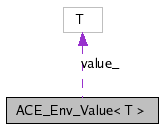# ACE_Env_Value< T > Class Template Reference

Environment Variable Value. More...

`#include <Env_Value_T.h>`

Collaboration diagram for ACE_Env_Value< T >:[legend]

List of all members.

## Public Member Functions

ACE_Env_Value (void)
ACE_Env_Value (const ACE_TCHAR *varname, const T &vardefault)
Constructor that calls <open>.
~ACE_Env_Value (void)
Destroy the value.
operator T (void)
Returns the value as type T.
void open (const ACE_TCHAR *varname, const T &defval)
const ACE_TCHARvarname (void) const
Returns the name of the variable being tracked.

## Private Member Functions

ACE_Env_Value (const ACE_Env_Value< T > &)
Disallow copying and assignment.
ACE_Env_Value< T > operator= (const ACE_Env_Value< T > &)
void fetch_value (void)

## Private Attributes

const ACE_TCHARvarname_
value_

## Detailed Description

### template<class T> class ACE_Env_Value< T >

Environment Variable Value.

Reads a variable from the user environment, providing a default value.

## Constructor & Destructor Documentation

template<class T >
 ACE_Env_Value< T >::ACE_Env_Value ( void ) ` [inline]`

Default constructor which isn't bound to a specific environment variable name or a default value. Before being useful it must <open>'d.

template<class T >
 ACE_Env_Value< T >::ACE_Env_Value ( const ACE_TCHAR * varname, const T & vardefault ) ` [inline]`

Constructor that calls <open>.

template<class T >
 ACE_Env_Value< T >::~ACE_Env_Value ( void ) ` [inline]`

Destroy the value.

template<class T >
 ACE_Env_Value< T >::ACE_Env_Value ( const ACE_Env_Value< T > & ) ` [private]`

Disallow copying and assignment.

## Member Function Documentation

template<class T >
 void ACE_Env_Value< T >::fetch_value ( void ) ` [inline, private]`

template<class T >
 void ACE_Env_Value< T >::open ( const ACE_TCHAR * varname, const T & defval ) ` [inline]`

The constructor, read varname from the environment, using defval as its value if it is not defined.

template<class T >
 ACE_Env_Value< T >::operator T ( void ) ` [inline]`

Returns the value as type T.

template<class T >
 ACE_Env_Value ACE_Env_Value< T >::operator= ( const ACE_Env_Value< T > & ) ` [private]`

template<class T >
 const ACE_TCHAR * ACE_Env_Value< T >::varname ( void ) const` [inline]`

Returns the name of the variable being tracked.

## Member Data Documentation

template<class T >
 T ACE_Env_Value< T >::value_` [private]`

template<class T >
 const ACE_TCHAR* ACE_Env_Value< T >::varname_` [private]`

The documentation for this class was generated from the following files:

Generated on Tue Apr 14 11:11:20 2009 for ACE by1.5.8